Successfully reported this slideshow.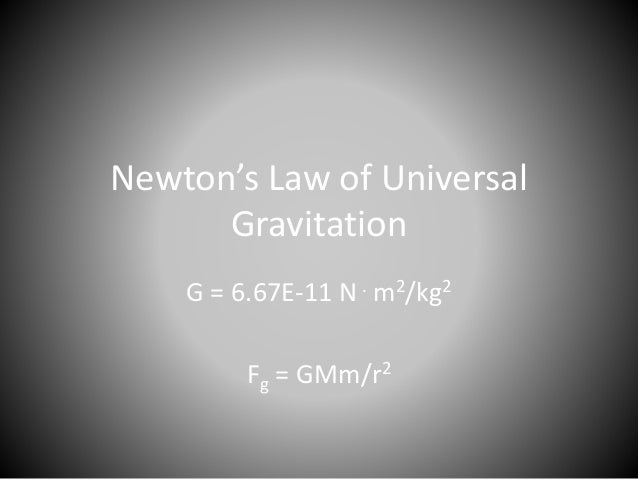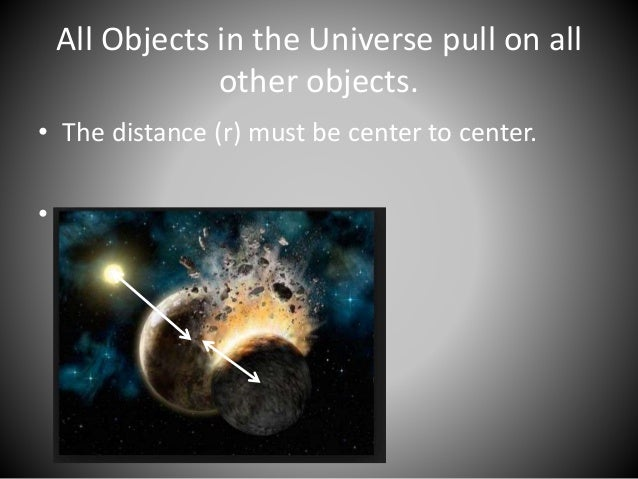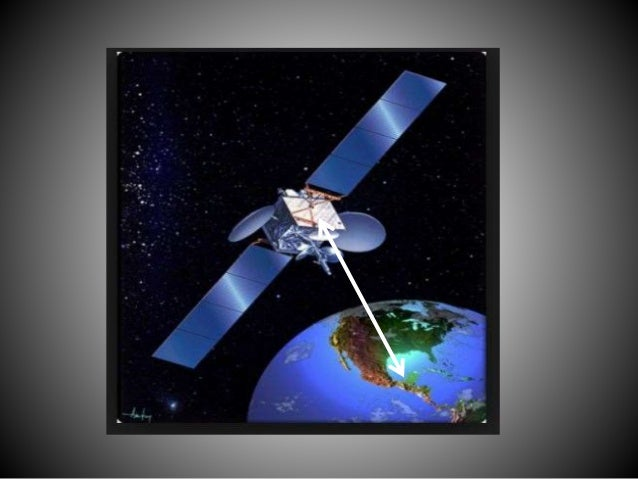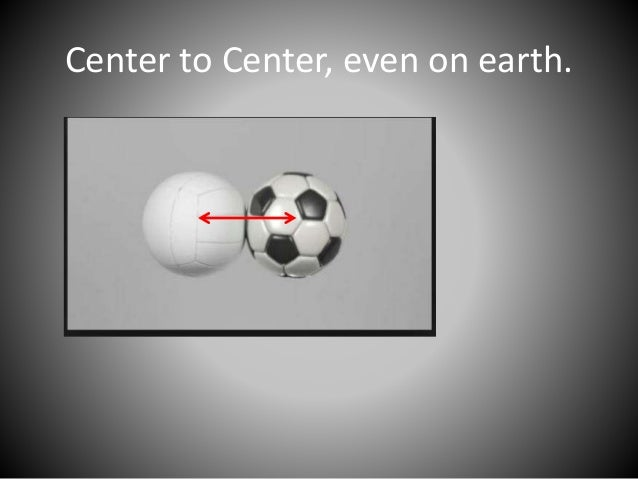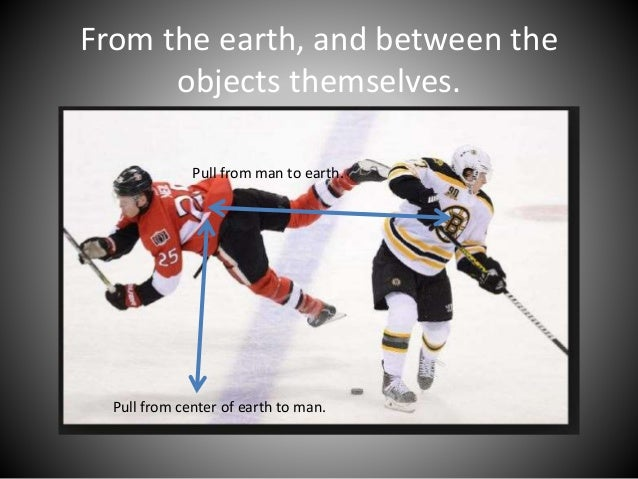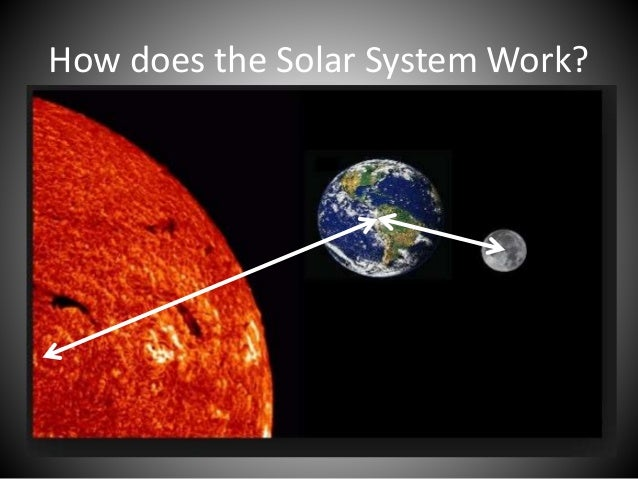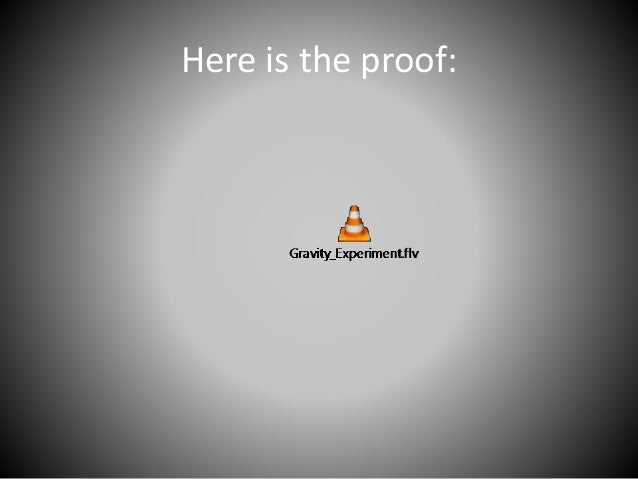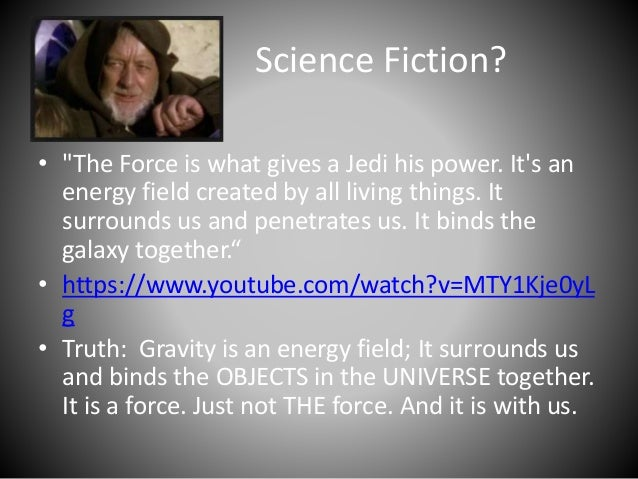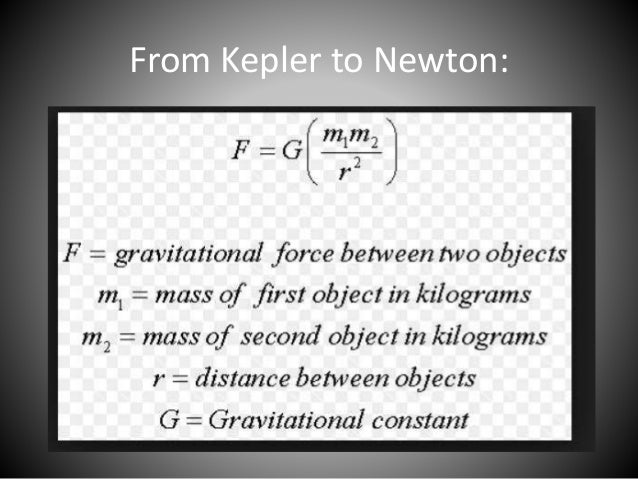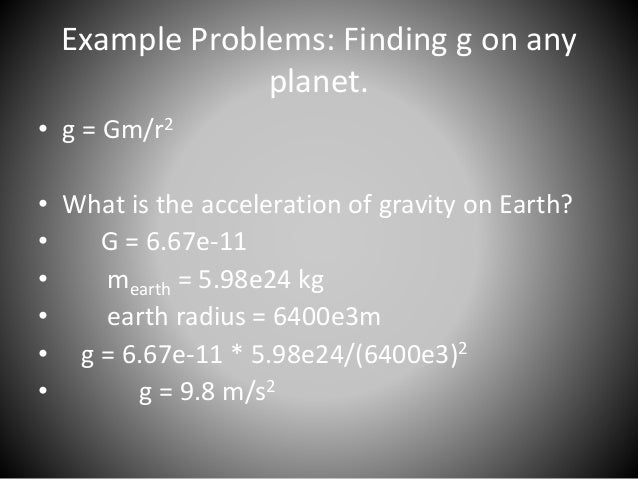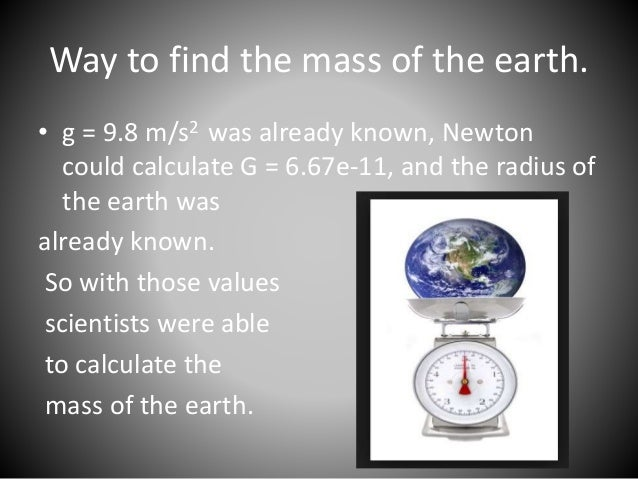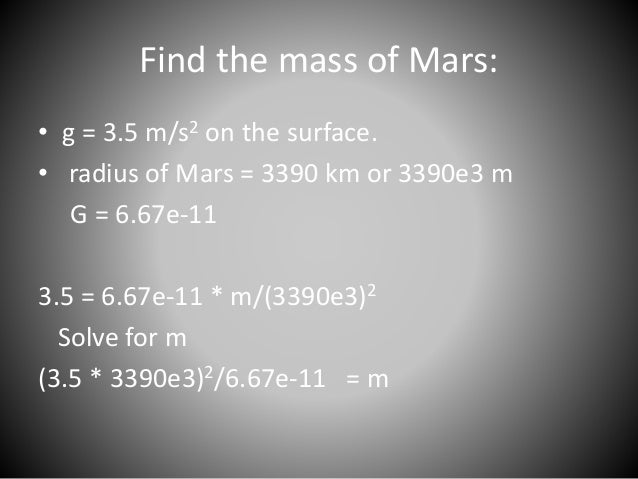Upcoming SlideShare
×

# Ch 2 -newton’s law of universal gravitation

A limited math presentation of the concept of gravitational force for a high school astronomy class.

• Full Name
Comment goes here.

Are you sure you want to Yes No• Be the first to comment

### Ch 2 -newton’s law of universal gravitation

1. 1. Newton’s Law of Universal Gravitation G = 6.67E-11 N. m2/kg2 Fg = GMm/r2
2. 2. All Objects in the Universe pull on all other objects. • The distance (r) must be center to center. •
3. 3. Center to Center, even on earth.
4. 4. From the earth, and between the objects themselves. Pull from man to earth. Pull from center of earth to man.
5. 5. How does the Solar System Work?
6. 6. Here is the proof:
7. 7. Science Fiction? • "The Force is what gives a Jedi his power. It's an energy field created by all living things. It surrounds us and penetrates us. It binds the galaxy together.“ • https://www.youtube.com/watch?v=MTY1Kje0yL g • Truth: Gravity is an energy field; It surrounds us and binds the OBJECTS in the UNIVERSE together. It is a force. Just not THE force. And it is with us.
8. 8. From Kepler to Newton:
9. 9. Example Problems: Finding g on any planet. • g = Gm/r2 • What is the acceleration of gravity on Earth? • G = 6.67e-11 • mearth = 5.98e24 kg • earth radius = 6400e3m • g = 6.67e-11 * 5.98e24/(6400e3)2 • g = 9.8 m/s2
10. 10. Way to find the mass of the earth. • g = 9.8 m/s2 was already known, Newton could calculate G = 6.67e-11, and the radius of the earth was already known. So with those values scientists were able to calculate the mass of the earth.
11. 11. Find the mass of Mars: • g = 3.5 m/s2 on the surface. • radius of Mars = 3390 km or 3390e3 m G = 6.67e-11 3.5 = 6.67e-11 * m/(3390e3)2 Solve for m (3.5 * 3390e3)2/6.67e-11 = m